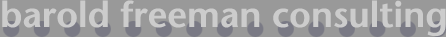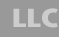### On the Accuracy of Samples1

The question often arises -- "How accurate is my sample?" The answer to this question is trickier than it sounds, since the level of accuracy will vary from question to question within a survey. Since the variance is different from one survey question to another, the survey's precision will vary from item to item.

The precision of a survey depends upon the variance of the responses to the question of interest, the level of confidence used, and the sample size. When the sample represents a significant proportion (experts say this can be anywhere between 2%-10%) of the population, this too must be taken into account when computing precision.

The approach often used by pollsters and researchers in reporting the precision of nationally representative surveys is a conservative approach; it assumes the "worst case." The worst case is represented by dichotomous data (0-1, yes-no) with maximum variability (50/50 distribution of responses). This approach is popular because it is simple. The first link below uses this approach to calculate the relative margin of error for such data. The second link below computes a measure of absolute precision for continuous data. This requires an estimate of  the mean and variabililty of the relevant population.

1. Paraphrased from Dr. Brian Blake's course materials for Psychology 512, Cleveland State University